# p5.js 3D图形-立方体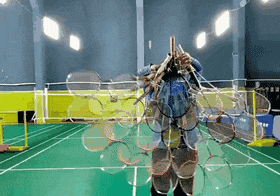# 立方体的基础用法

`p5.js` 里使用 `box()` 方法可以创建立方体。

## 基础语法说明

``````box([width], [height], [depth], [detailX], [detailY])

• `width`：立方体的宽度（选填），默认值是 50。
• `height`：立方体的高度（选填）。
• `depth`：立方体的深度（选填）。
• `detailX`：一个用于指定立方体在x轴方向上的细分级别的数字，数值越大，立方体的表面越平滑。（选填）
• `detailY`：一个用于指定立方体在y轴方向上的细分级别的数字，数值越大，立方体的表面越平滑。（选填）

1. 3个参数都不传的情况：它们的值默认为50。
2. 只传 `width` 的情况：`height``depth` 都会跟着使用 `width` 的值。
3. 传了 `width``height` 的情况：`depth` 会使用 `height` 的值。
4. 3个参数都有传的情况：各自使用各自的值。

## 动手试试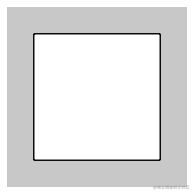``````<script>
function setup() {
createCanvas(200, 200, WEBGL) // 创建 200 * 200 的画布
background(200) // 设置画布背景色（灰色）
box(100) // 创建立方体
}
</script>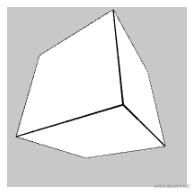``````<script>
function setup() {
createCanvas(200, 200, WEBGL) // 创建 200 * 200 的画布
background(200) // 设置画布背景色（灰色）
// 旋转角度
rotateX(10)
rotateY(10)
box(100) // 创建立方体
}
</script>

## 设置样式

### 填充色 fill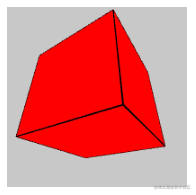``````<script>
function setup() {
createCanvas(200, 200, WEBGL) // 创建 200 * 200 的画布
background(200) // 设置画布背景色（灰色）

// 旋转角度
rotateX(10)
rotateY(10)

// 填充色
fill(255, 0, 0)

box(100) // 创建立方体
}
</script>

### 不使用填充颜色

`box()` 的默认填充色是白色，如果你不需要填充色，可以使用 `noFill()` 方法进行修改。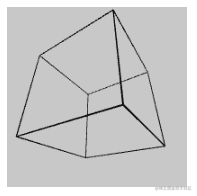``````<script>
function setup() {
createCanvas(200, 200, WEBGL) // 创建 200 * 200 的画布
background(200) // 设置画布背景色（灰色）

// 旋转角度
rotateX(10)
rotateY(10)

// 不使用填充颜色
noFill()

box(100) // 创建立方体
}
</script>

### 描边颜色 stroke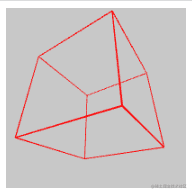``````<script>
function setup() {
createCanvas(200, 200, WEBGL)
background(200)

noFill()
stroke(255, 0, 0) // 设置红色边框颜色

rotateX(10)
rotateY(10)

box(100)
}
</script>

### 设置边框宽度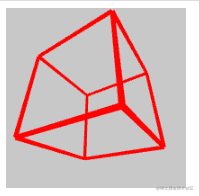``````<script>
function setup() {
createCanvas(200, 200, WEBGL)
background(200)

noFill()
stroke(255, 0, 0) // 设置红色边框颜色
strokeWeight(4) // 设置边框宽度

rotateX(10)
rotateY(10)

box(100)
}
</script>

### 纹理

1. 加载纹理资源（图片或者视频）
2. 设置纹理
3. 创建立方体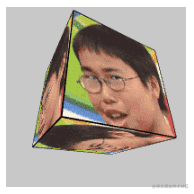``````<script>
let myTexture = null

}

function setup() {
createCanvas(200, 200, WEBGL)
background(200)

rotateX(0.5)
rotateY(0.5)

texture(myTexture) // 设置纹理

box(100) // 创建立方体
}
</script>

### 光照效果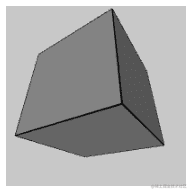``````<script>
function setup() {
createCanvas(200, 200, WEBGL)
background(200)

rotateX(10)
rotateY(10)

ambientLight(255) // 设置环境光照
directionalLight(255, 255, 255, 0, 0, -1) // 设置定向光照

box(100)
}
</script>

## 动画

### 旋转动画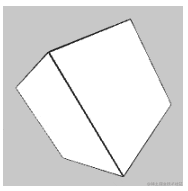``````<script>
function setup() {
createCanvas(200, 200, WEBGL)
}

function draw() {
// 每次刷新都要重新填充画布颜色，不然会留下上一次绘制的立方体
background(200)

// 旋转
rotateX(frameCount * 0.01)
rotateY(frameCount * 0.01)
rotateZ(frameCount * 0.01)

// 绘制立方体
box(100)
}
</script>

### gif 贴图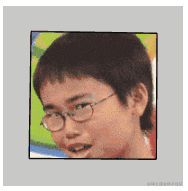``````<script>
let myTexture = null

}

function setup() {
createCanvas(200, 200, WEBGL)
}

function draw() {
background(200)

rotateX(frameCount * 0.01)
rotateY(frameCount * 0.01)
rotateZ(frameCount * 0.01)

// 设置纹理贴图
texture(myTexture)
box(100)
}
</script>

### 视频纹理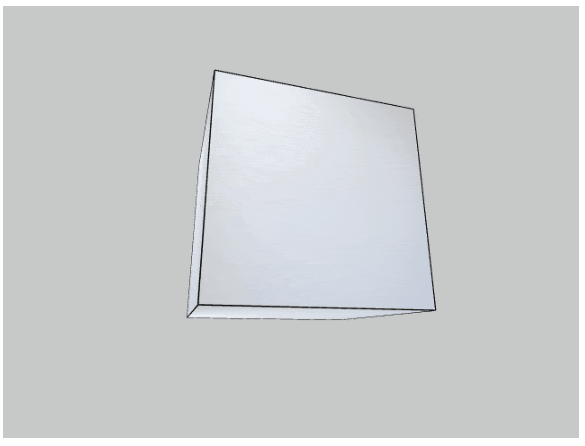``````<script>
let video = null

video = createVideo('video.mp4') // 加载 mp4
video.hide() // 隐藏视频元素
}

function setup() {
createCanvas(640, 480, WEBGL)
video.loop() // 循环播放
video.volume(0) // 设置音量
}

function draw() {
background(200)

rotateX(frameCount * 0.01)
rotateY(frameCount * 0.01)

// 设置视频纹理
texture(video)
box(200)
}
</script>

• `video.loop()`：循环播放。
• `video.volume()`：设置视频音量，取值范围是 `0 ~ 1`

# 小案例

`p5.js` 是一个偏艺术类的 `canvas` 库，我们已经掌握了 `box()` 基础用法创建出立方体，接下来再理解几个小案例应该就有能力自己去实现一些特效了。非常适合在掘金整活~

## 案例1：Rotate Push Pop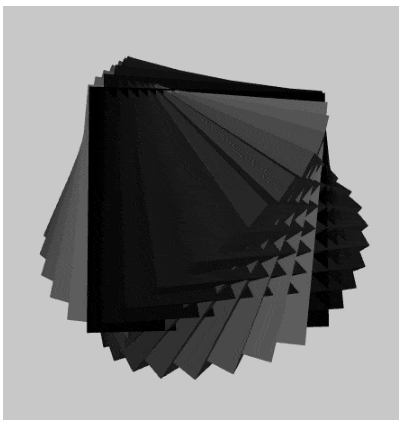``````<script>
let a = 0
let offset = Math.PI / 24
let num = 12

function setup() {
createCanvas(440, 460, WEBGL)
noStroke()
}

function draw() {
lights()
background(200, 200, 200)

for(let i = 0; i < num; i++) {
// map 映射
let gray = map(i, 0, num - 1, 0, 255)
push()
fill(gray)
rotateY(a + offset * i)
rotateX(a / 2 + offset * i)
box(200)
pop()
}

a += 0.01
}
</script>

## 案例2：运动的立方体们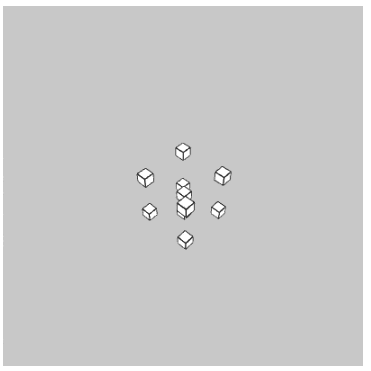``````<script>
function setup() {
createCanvas(400, 400, WEBGL)
}

let letter = [
[0, 0, 0],
[0, 0, 50],
[25, 0, 25],
[0, 50, 0],
[0, 50, 50],
[25, 25, 25],
[50, 0, 0],
[50, 0, 50],
[50, 50, 0],
[50, 50, 50]
]

function draw() {
background(200)
rotateX(frameCount * 0.01)
rotateY(frameCount * 0.01)
for (let i = 0; i < letter.length; i++) {
push()
translate(letter[i], letter[i], letter[i])
box(10)
pop()
}
}
</script>

`letter` 创建了一堆坐标点，他们记录了立方体们的位置。在 `draw()` 里不断的改变他们的位置。

## 案例3：排列立方体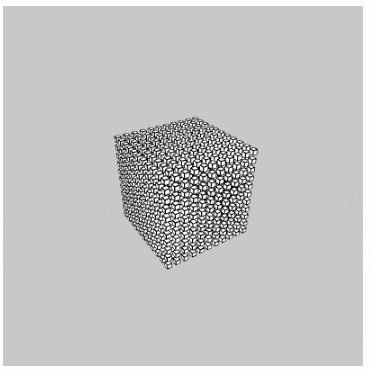``````<script>
function setup() {
createCanvas(400, 400, WEBGL)
}

function draw() {
background(200);
rotateX(frameCount * 0.01);
rotateY(frameCount * 0.01);
for (let x = -50; x <= 50; x += 10) {
for (let y = -50; y <= 50; y += 10) {
for (let z = -50; z <= 50; z += 10) {
push();
translate(x, y, z);
box(5);
pop();
}
}
}
}
</script>

## 案例4：还是立方体，我不知道怎么起名了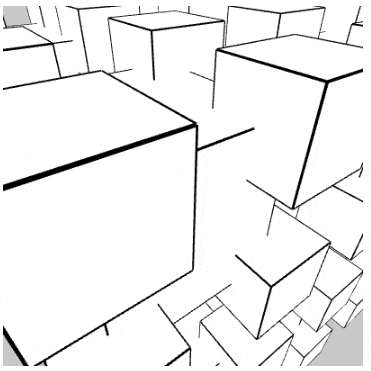``````<script>
function setup() {
createCanvas(400, 400, WEBGL)
}

function fractalBox(size, level) {
box(size)
if (level > 1) {
level--
push()
translate(-size/2, -size/2, -size/2)
fractalBox(size/2, level)
pop()

push()
translate(-size/2, -size/2, size/2)
fractalBox(size/2, level)
pop()

push()
translate(-size/2, size/2, -size/2)
fractalBox(size/2, level)
pop()

push()
translate(-size/2, size/2, size/2)
fractalBox(size/2, level)
pop()

push()
translate(size/2, -size/2, -size/2)
fractalBox(size/2, level)
pop()

push()
translate(size/2, -size/2, size/2)
fractalBox(size/2, level)
pop()

push()
translate(size/2, size/2, -size/2)
fractalBox(size/2, level)
pop()

push()
translate(size/2, size/2, size/2)
fractalBox(size/2, level)
pop()
}
}

function draw() {
background(200)
rotateX(frameCount * 0.01)
rotateY(frameCount * 0.01)
fractalBox(200, 3)
}
</script>